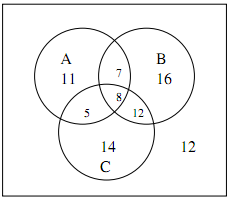## Construct a venn diagram, Mathematics

Assignment Help:

In a survey of 85 people this is found that 31 want to drink milk 43 like coffee and 39 wish tea.  As well 13 want both milk and tea, 15 like milk & coffee, 20 like tea and coffee and 12 like none of the three drinks.  Find out the number of people who want all the three drinks.  Display the answer by using Venn Diagram.

Ans:  Let A, B and C is set of people who wish to drink milk, take coffee and take tea correspondingly. So |A| = 31, |B| = 43, |C| = 39, |A∩B| = 15, |A∩C| = 13, |B∩C| = 20, |A∪B∪C| = 85 - 12 = 73. Hence, |A∩B∩C| = 73 + (15 + 13 + 20) - (31 + 43 + 39) = 8.24x+7=3x+10

#### Number of permutations of ''n'' dissimilar things , Finding the numbe...

Finding the number of Permutations of 'n' dissimilar things taken 'r' at a time:  After looking at the definition of permutations, we look at how to evolve a

#### Product and brand management, core competency vs diversification

core competency vs diversification

#### Which a dog is their favorite type of pet, The students at Norton School we...

The students at Norton School were asked to name their favorite type of pet. Of the 430 students surveyed, 258 said in that their favorite type of pet was a dog. Assume that only 1

#### Differential equation, Suppose a fluid (say, water) occupies a domain D? R^...

Suppose a fluid (say, water) occupies a domain D? R^(3 ) and has velocity field V=V(x, t). A substance (say, a day) is suspended into the fluid and will be transported by the fluid

#### Natural numbers, To begin with we have counting numbers. These ...

To begin with we have counting numbers. These numbers are also known as natural numbers and are denoted by a symbol 'N'. These numbers are obtai

what is answer ? + 5=10+5

#### Example of repeated eigenvalues, Illustration : Solve the following IVP. ...

Illustration : Solve the following IVP. Solution: First get the eigenvalues for the system. = l 2 - 10 l+ 25 = (l- 5) 2 l 1,2 = 5 Therefore, we got a

#### Prove that x2 + y2 - 8x - 10y +39 = 0, If the points (5, 4) and (x, y) are ...

If the points (5, 4) and (x, y) are equidistant from the point (4, 5), prove that x 2 + y 2 - 8x - 10y +39 = 0. Ans :   AP = PB AP 2 = PB 2 (5 - 4) 2 + (4 - 5) 2 = (x

#### Arithmetic progression, the radii of circular base of right circular cylind...

the radii of circular base of right circular cylinder and cone are in the ratio of 3:4 and their height are in the ratio of the 2:3 what is the ratio of their volume?